# Output Feedback

Jump to: navigation, search
 Prev: State Feedback Chapter 7 - Output Feedback Next: Transfer Functions

In the last chapter we considered the use of state feedback to modify the dynamics of a system through feedback. In many applications, it is not practical to measure all of the states directly and we can measure only a small number of outputs (corresponding to the sensors that are available). In this chapter we show how to use output feedback to modify the dynamics of the system, through the use of state estimators (also called "observers"). We introduce the concept of observability and show that if a system is observable, itis possible to recover the state from measurements of the inputs andoutputs to the system.

## Textbook Contents

• 1. Observability
• 2. State Estimation
• 3. Control Using Estimated State
• 4. Kalman Filtering*
• 5. State Space Control Systems
• 6. Further Reading
• Exercises

## Chapter Summary

This chapter describes how to estimate the state of a system through measurements of its inputs and outputs:

1. A linear system with dynamicsis said to be observable if we can determine the state of the system through measurements of the inputand the output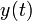over a time interval.

2. The observability matrix for a linear system is given byA linear system is observable if and only if the observability matrix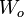is full rank. Systems that are not reachable have "hidden" states that cannot be determined by looking at the inputs and outputs.

3. A linear system of the form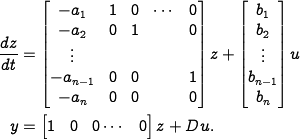is said to be in observable canonical form. A system in this form is always observable and has a characteristic polynomial given by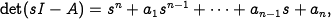An observable linear system can be transformed into observable canonical form through the use of a coordinate transformation.

4. An observer is a dynamical system that estimates the state of another system through measurement of inputs and outputs. For a linear system, the observer given by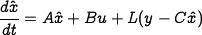generates an estimate of the state that converges to the actual state ifis has eigenvalues with negative real part. If a system is observable, then there exists a an observer gain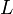such that the observer error is governed by a linear differential equation with an arbitrary characteristic polynomial. Hence the eigenvalues of the error dynamics for an observable linear system can be placed arbitrarily through the use of an appropriate observer gain.

5. A state feedback controller and linear observer can be combined to form a stabilizing controller for a reachable and observable linear system by using the estimate of the state in the feedback control law. The resulting controller is given by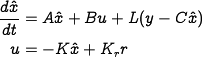6. A discrete time, linear process with noise is given bywhere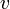is a vector, white, Gaussian random process with mean 0, autocovariance,is a white, Guassian random process with mean 0, variance. We take the initial condition to be random with mean 0 and covariance. The optimal estimator is given by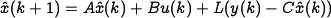where the observer gain satisfies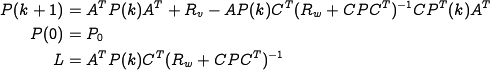This estimator is an example of a Kalman filter.